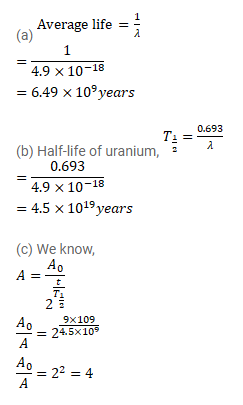# The decay constant of

Question:

The decay constant of ${ }^{235} \mathrm{U}$ is $4.9 \times 10^{-18} \mathrm{~s}^{-1}$.

(a) What is the average- life of ${ }^{235} \mathrm{U}$ ?

(b) What is the half-life of ${ }^{235} \mathrm{U}$ ?

(c) By what factor does the activity of $a^{235} \mathrm{U}$ sample decrease in $9 \times 10^{8}$ years?

Solution: